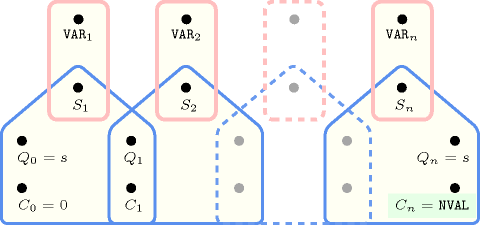## 5.172. group

Origin

CHIP

Constraint

$\mathrm{𝚐𝚛𝚘𝚞𝚙}\left(\begin{array}{c}\mathrm{𝙽𝙶𝚁𝙾𝚄𝙿},\hfill \\ \mathrm{𝙼𝙸𝙽}_\mathrm{𝚂𝙸𝚉𝙴},\hfill \\ \mathrm{𝙼𝙰𝚇}_\mathrm{𝚂𝙸𝚉𝙴},\hfill \\ \mathrm{𝙼𝙸𝙽}_\mathrm{𝙳𝙸𝚂𝚃},\hfill \\ \mathrm{𝙼𝙰𝚇}_\mathrm{𝙳𝙸𝚂𝚃},\hfill \\ \mathrm{𝙽𝚅𝙰𝙻},\hfill \\ \mathrm{𝚅𝙰𝚁𝙸𝙰𝙱𝙻𝙴𝚂},\hfill \\ \mathrm{𝚅𝙰𝙻𝚄𝙴𝚂}\hfill \end{array}\right)$

Arguments
 $\mathrm{𝙽𝙶𝚁𝙾𝚄𝙿}$ $\mathrm{𝚍𝚟𝚊𝚛}$ $\mathrm{𝙼𝙸𝙽}_\mathrm{𝚂𝙸𝚉𝙴}$ $\mathrm{𝚍𝚟𝚊𝚛}$ $\mathrm{𝙼𝙰𝚇}_\mathrm{𝚂𝙸𝚉𝙴}$ $\mathrm{𝚍𝚟𝚊𝚛}$ $\mathrm{𝙼𝙸𝙽}_\mathrm{𝙳𝙸𝚂𝚃}$ $\mathrm{𝚍𝚟𝚊𝚛}$ $\mathrm{𝙼𝙰𝚇}_\mathrm{𝙳𝙸𝚂𝚃}$ $\mathrm{𝚍𝚟𝚊𝚛}$ $\mathrm{𝙽𝚅𝙰𝙻}$ $\mathrm{𝚍𝚟𝚊𝚛}$ $\mathrm{𝚅𝙰𝚁𝙸𝙰𝙱𝙻𝙴𝚂}$ $\mathrm{𝚌𝚘𝚕𝚕𝚎𝚌𝚝𝚒𝚘𝚗}\left(\mathrm{𝚟𝚊𝚛}-\mathrm{𝚍𝚟𝚊𝚛}\right)$ $\mathrm{𝚅𝙰𝙻𝚄𝙴𝚂}$ $\mathrm{𝚌𝚘𝚕𝚕𝚎𝚌𝚝𝚒𝚘𝚗}\left(\mathrm{𝚟𝚊𝚕}-\mathrm{𝚒𝚗𝚝}\right)$
Restrictions
 $\mathrm{𝙽𝙶𝚁𝙾𝚄𝙿}\ge 0$ $\mathrm{𝙼𝙸𝙽}_\mathrm{𝚂𝙸𝚉𝙴}\ge 0$ $\mathrm{𝙼𝙰𝚇}_\mathrm{𝚂𝙸𝚉𝙴}\ge \mathrm{𝙼𝙸𝙽}_\mathrm{𝚂𝙸𝚉𝙴}$ $\mathrm{𝙼𝙸𝙽}_\mathrm{𝙳𝙸𝚂𝚃}\ge 0$ $\mathrm{𝙼𝙰𝚇}_\mathrm{𝙳𝙸𝚂𝚃}\ge \mathrm{𝙼𝙸𝙽}_\mathrm{𝙳𝙸𝚂𝚃}$ $\mathrm{𝙼𝙰𝚇}_\mathrm{𝙳𝙸𝚂𝚃}\le |\mathrm{𝚅𝙰𝚁𝙸𝙰𝙱𝙻𝙴𝚂}|$ $\mathrm{𝙽𝚅𝙰𝙻}\ge \mathrm{𝙼𝙰𝚇}_\mathrm{𝚂𝙸𝚉𝙴}$ $\mathrm{𝙽𝚅𝙰𝙻}\ge \mathrm{𝙽𝙶𝚁𝙾𝚄𝙿}$ $\mathrm{𝙽𝚅𝙰𝙻}\le |\mathrm{𝚅𝙰𝚁𝙸𝙰𝙱𝙻𝙴𝚂}|$ $\mathrm{𝚛𝚎𝚚𝚞𝚒𝚛𝚎𝚍}$$\left(\mathrm{𝚅𝙰𝚁𝙸𝙰𝙱𝙻𝙴𝚂},\mathrm{𝚟𝚊𝚛}\right)$ $\mathrm{𝚛𝚎𝚚𝚞𝚒𝚛𝚎𝚍}$$\left(\mathrm{𝚅𝙰𝙻𝚄𝙴𝚂},\mathrm{𝚟𝚊𝚕}\right)$ $\mathrm{𝚍𝚒𝚜𝚝𝚒𝚗𝚌𝚝}$$\left(\mathrm{𝚅𝙰𝙻𝚄𝙴𝚂},\mathrm{𝚟𝚊𝚕}\right)$
Purpose

Let $n$ be the number of variables of the collection $\mathrm{𝚅𝙰𝚁𝙸𝙰𝙱𝙻𝙴𝚂}$. Let ${X}_{i},{X}_{i+1},\cdots ,{X}_{j}$ $\left(1\le i\le j\le n\right)$ be consecutive variables of the collection of variables $\mathrm{𝚅𝙰𝚁𝙸𝙰𝙱𝙻𝙴𝚂}$ such that all the following conditions simultaneously apply:

• All variables ${X}_{i},\cdots ,{X}_{j}$ take their value in the set of values $\mathrm{𝚅𝙰𝙻𝚄𝙴𝚂}$,

• $i=1$ or ${X}_{i-1}$ does not take a value in $\mathrm{𝚅𝙰𝙻𝚄𝙴𝚂}$,

• $j=n$ or ${X}_{j+1}$ does not take a value in $\mathrm{𝚅𝙰𝙻𝚄𝙴𝚂}$.

We call such a sequence of variables a group. Similarly an anti-group is a maximum sequence of variables that are not assigned any value from $\mathrm{𝚅𝙰𝙻𝚄𝙴𝚂}$. The constraint $\mathrm{𝚐𝚛𝚘𝚞𝚙}$ is true if all the following conditions hold:

• There are exactly $\mathrm{𝙽𝙶𝚁𝙾𝚄𝙿}$ groups of variables,

• $\mathrm{𝙼𝙸𝙽}_\mathrm{𝚂𝙸𝚉𝙴}$ is the number of variables of the smallest group,

• $\mathrm{𝙼𝙰𝚇}_\mathrm{𝚂𝙸𝚉𝙴}$ is the number of variables of the largest group,

• $\mathrm{𝙼𝙸𝙽}_\mathrm{𝙳𝙸𝚂𝚃}$ is the number of variables of the smallest anti-group,

• $\mathrm{𝙼𝙰𝚇}_\mathrm{𝙳𝙸𝚂𝚃}$ is the number of variables of the largest anti-group,

• $\mathrm{𝙽𝚅𝙰𝙻}$ is the number of variables that take their value in the set of values $\mathrm{𝚅𝙰𝙻𝚄𝙴𝚂}$.

Example
$\left(2,1,2,2,4,3,〈2,8,1,7,4,5,1,1,1〉,〈0,2,4,6,8〉\right)$

Given the fact that groups are formed by even values in $\left\{0,2,4,6,8\right\}$ (i.e., values expressed by the $\mathrm{𝚅𝙰𝙻𝚄𝙴𝚂}$ collection), the $\mathrm{𝚐𝚛𝚘𝚞𝚙}$ constraint holds since:

• Its first argument, $\mathrm{𝙽𝙶𝚁𝙾𝚄𝙿}$, is set to value 2 since the sequence $281745111$ contains two groups of even values (i.e., group $28$ and group 4).

• Its second argument, $\mathrm{𝙼𝙸𝙽}_\mathrm{𝚂𝙸𝚉𝙴}$, is set to value 1 since the smallest group of even values involves only a single value (i.e., value 4).

• Its third argument, $\mathrm{𝙼𝙰𝚇}_\mathrm{𝚂𝙸𝚉𝙴}$, is set to value 2 since the largest group of even values involves two values (i.e., group $28$).

• Its fourth argument, $\mathrm{𝙼𝙸𝙽}_\mathrm{𝙳𝙸𝚂𝚃}$, is set to value 2 since the smallest anti-group involves two values (i.e., anti-group $17$).

• Its fifth argument, $\mathrm{𝙼𝙰𝚇}_\mathrm{𝙳𝙸𝚂𝚃}$, is set to value 4 since the largest anti-group involves four values (i.e., anti-group $5111$).

• Its sixth argument, $\mathrm{𝙽𝚅𝙰𝙻}$, is set to value 3 since the total number of even values of the sequence $281745111$ is equal to 3 (i.e., values 2, 8 and 4).

All solutions

Figure 5.172.1 gives all solutions to the following non ground instance of the $\mathrm{𝚐𝚛𝚘𝚞𝚙}$ constraint: $\mathrm{𝙽𝙶𝚁𝙾𝚄𝙿}\in \left[2,3\right]$, $\mathrm{𝙼𝙸𝙽}_\mathrm{𝚂𝙸𝚉𝙴}\in \left[3,4\right]$, $\mathrm{𝙼𝙰𝚇}_\mathrm{𝚂𝙸𝚉𝙴}\in \left[3,5\right]$, $\mathrm{𝙼𝙸𝙽}_\mathrm{𝙳𝙸𝚂𝚃}\in \left[1,2\right]$, $\mathrm{𝙼𝙰𝚇}_\mathrm{𝙳𝙸𝚂𝚃}\in \left[1,2\right]$, $\mathrm{𝙽𝚅𝙰𝙻}\in \left[5,6\right]$, ${V}_{1}\in \left[0,1\right]$, ${V}_{2}\in \left[0,1\right]$, ${V}_{3}\in \left[0,1\right]$, ${V}_{4}\in \left[0,1\right]$, ${V}_{5}\in \left[0,1\right]$, ${V}_{6}\in \left[0,1\right]$, ${V}_{7}\in \left[0,1\right]$, ${V}_{8}\in \left[0,1\right]$, ${V}_{9}\in \left[0,1\right]$, $\mathrm{𝚐𝚛𝚘𝚞𝚙}$$\left(\mathrm{𝙽𝙶𝚁𝙾𝚄𝙿},\mathrm{𝙼𝙸𝙽}_\mathrm{𝚂𝙸𝚉𝙴},\mathrm{𝙼𝙰𝚇}_\mathrm{𝚂𝙸𝚉𝙴},\mathrm{𝙼𝙸𝙽}_\mathrm{𝙳𝙸𝚂𝚃},\mathrm{𝙼𝙰𝚇}_\mathrm{𝙳𝙸𝚂𝚃},\mathrm{𝙽𝚅𝙰𝙻},$ $〈{V}_{1},{V}_{2},{V}_{3},{V}_{4},{V}_{5},{V}_{6},{V}_{7},{V}_{8},{V}_{9}〉,〈1〉\right)$,

##### Figure 5.172.1. All solutions corresponding to the non ground example of the $\mathrm{𝚐𝚛𝚘𝚞𝚙}$ constraint of the All solutions slot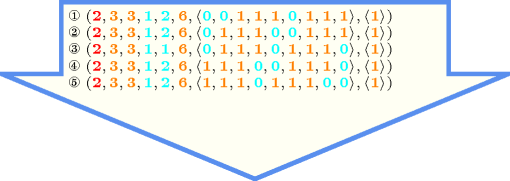Typical
 $\mathrm{𝙽𝙶𝚁𝙾𝚄𝙿}>0$ $\mathrm{𝙼𝙸𝙽}_\mathrm{𝚂𝙸𝚉𝙴}>0$ $\mathrm{𝙼𝙰𝚇}_\mathrm{𝚂𝙸𝚉𝙴}>\mathrm{𝙼𝙸𝙽}_\mathrm{𝚂𝙸𝚉𝙴}$ $\mathrm{𝙼𝙸𝙽}_\mathrm{𝙳𝙸𝚂𝚃}>0$ $\mathrm{𝙼𝙰𝚇}_\mathrm{𝙳𝙸𝚂𝚃}>\mathrm{𝙼𝙸𝙽}_\mathrm{𝙳𝙸𝚂𝚃}$ $\mathrm{𝙼𝙰𝚇}_\mathrm{𝙳𝙸𝚂𝚃}<|\mathrm{𝚅𝙰𝚁𝙸𝙰𝙱𝙻𝙴𝚂}|$ $\mathrm{𝙽𝚅𝙰𝙻}>\mathrm{𝙼𝙰𝚇}_\mathrm{𝚂𝙸𝚉𝙴}$ $\mathrm{𝙽𝚅𝙰𝙻}>\mathrm{𝙽𝙶𝚁𝙾𝚄𝙿}$ $\mathrm{𝙽𝚅𝙰𝙻}<|\mathrm{𝚅𝙰𝚁𝙸𝙰𝙱𝙻𝙴𝚂}|$ $|\mathrm{𝚅𝙰𝚁𝙸𝙰𝙱𝙻𝙴𝚂}|>1$ $\mathrm{𝚛𝚊𝚗𝚐𝚎}$$\left(\mathrm{𝚅𝙰𝚁𝙸𝙰𝙱𝙻𝙴𝚂}.\mathrm{𝚟𝚊𝚛}\right)>1$ $|\mathrm{𝚅𝙰𝙻𝚄𝙴𝚂}|>0$ $|\mathrm{𝚅𝙰𝚁𝙸𝙰𝙱𝙻𝙴𝚂}|>|\mathrm{𝚅𝙰𝙻𝚄𝙴𝚂}|$
Symmetries
• Items of $\mathrm{𝚅𝙰𝚁𝙸𝙰𝙱𝙻𝙴𝚂}$ can be reversed.

• Items of $\mathrm{𝚅𝙰𝙻𝚄𝙴𝚂}$ are permutable.

• An occurrence of a value of $\mathrm{𝚅𝙰𝚁𝙸𝙰𝙱𝙻𝙴𝚂}.\mathrm{𝚟𝚊𝚛}$ that belongs to $\mathrm{𝚅𝙰𝙻𝚄𝙴𝚂}.\mathrm{𝚟𝚊𝚕}$ (resp. does not belong to $\mathrm{𝚅𝙰𝙻𝚄𝙴𝚂}.\mathrm{𝚟𝚊𝚕}$) can be replaced by any other value in $\mathrm{𝚅𝙰𝙻𝚄𝙴𝚂}.\mathrm{𝚟𝚊𝚕}$ (resp. not in $\mathrm{𝚅𝙰𝙻𝚄𝙴𝚂}.\mathrm{𝚟𝚊𝚕}$).

Arg. properties
• Functional dependency: $\mathrm{𝙽𝙶𝚁𝙾𝚄𝙿}$ determined by $\mathrm{𝚅𝙰𝚁𝙸𝙰𝙱𝙻𝙴𝚂}$ and $\mathrm{𝚅𝙰𝙻𝚄𝙴𝚂}$.

• Functional dependency: $\mathrm{𝙼𝙸𝙽}_\mathrm{𝚂𝙸𝚉𝙴}$ determined by $\mathrm{𝚅𝙰𝚁𝙸𝙰𝙱𝙻𝙴𝚂}$ and $\mathrm{𝚅𝙰𝙻𝚄𝙴𝚂}$.

• Functional dependency: $\mathrm{𝙼𝙰𝚇}_\mathrm{𝚂𝙸𝚉𝙴}$ determined by $\mathrm{𝚅𝙰𝚁𝙸𝙰𝙱𝙻𝙴𝚂}$ and $\mathrm{𝚅𝙰𝙻𝚄𝙴𝚂}$.

• Functional dependency: $\mathrm{𝙼𝙸𝙽}_\mathrm{𝙳𝙸𝚂𝚃}$ determined by $\mathrm{𝚅𝙰𝚁𝙸𝙰𝙱𝙻𝙴𝚂}$ and $\mathrm{𝚅𝙰𝙻𝚄𝙴𝚂}$.

• Functional dependency: $\mathrm{𝙼𝙰𝚇}_\mathrm{𝙳𝙸𝚂𝚃}$ determined by $\mathrm{𝚅𝙰𝚁𝙸𝙰𝙱𝙻𝙴𝚂}$ and $\mathrm{𝚅𝙰𝙻𝚄𝙴𝚂}$.

• Functional dependency: $\mathrm{𝙽𝚅𝙰𝙻}$ determined by $\mathrm{𝚅𝙰𝚁𝙸𝙰𝙱𝙻𝙴𝚂}$ and $\mathrm{𝚅𝙰𝙻𝚄𝙴𝚂}$.

Usage

A typical use of the $\mathrm{𝚐𝚛𝚘𝚞𝚙}$ constraint in the context of timetabling is as follow: The value of the ${i}^{th}$ variable of the $\mathrm{𝚅𝙰𝚁𝙸𝙰𝙱𝙻𝙴𝚂}$ collection corresponds to the type of shift (i.e., night, morning, afternoon, rest) performed by a specific person on day $i$. A complete period of work is represented by the variables of the $\mathrm{𝚅𝙰𝚁𝙸𝙰𝙱𝙻𝙴𝚂}$ collection. In this context the $\mathrm{𝚐𝚛𝚘𝚞𝚙}$ constraint expresses for a person:

• The number of periods of consecutive night-shift during a complete period of work.

• The total number of night-shift during a complete period of work.

• The maximum number of allowed consecutive night-shift.

• The minimum number of days, which do not correspond to a night-shift, between two consecutive sequences of night-shift.

Remark

For this constraint we use the possibility to express directly more than one constraint on the parameters of the final graph we want to obtain. For more propagation, it is crucial to keep this in a single constraint, since strong relations relate the different parameters of a graph. This constraint is very similar to the $\mathrm{𝚐𝚛𝚘𝚞𝚙}$ constraint introduced in CHIP, except that here, the $\mathrm{𝙼𝙸𝙽}_\mathrm{𝙳𝙸𝚂𝚃}$ and $\mathrm{𝙼𝙰𝚇}_\mathrm{𝙳𝙸𝚂𝚃}$ constraints apply also for the two borders: we cannot start or end with a group of $k$ consecutive variables that take their values outside $\mathrm{𝚅𝙰𝙻𝚄𝙴𝚂}$ and such that $k$ is less than $\mathrm{𝙼𝙸𝙽}_\mathrm{𝙳𝙸𝚂𝚃}$ or $k$ is greater than $\mathrm{𝙼𝙰𝚇}_\mathrm{𝙳𝙸𝚂𝚃}$.

Keywords
Arc input(s)

$\mathrm{𝚅𝙰𝚁𝙸𝙰𝙱𝙻𝙴𝚂}$

Arc generator
 $\mathrm{𝑃𝐴𝑇𝐻}$$↦\mathrm{𝚌𝚘𝚕𝚕𝚎𝚌𝚝𝚒𝚘𝚗}\left(\mathrm{𝚟𝚊𝚛𝚒𝚊𝚋𝚕𝚎𝚜}\mathtt{1},\mathrm{𝚟𝚊𝚛𝚒𝚊𝚋𝚕𝚎𝚜}\mathtt{2}\right)$ $\mathrm{𝐿𝑂𝑂𝑃}$$↦\mathrm{𝚌𝚘𝚕𝚕𝚎𝚌𝚝𝚒𝚘𝚗}\left(\mathrm{𝚟𝚊𝚛𝚒𝚊𝚋𝚕𝚎𝚜}\mathtt{1},\mathrm{𝚟𝚊𝚛𝚒𝚊𝚋𝚕𝚎𝚜}\mathtt{2}\right)$

Arc arity
Arc constraint(s)
 $•$$\mathrm{𝚒𝚗}$$\left(\mathrm{𝚟𝚊𝚛𝚒𝚊𝚋𝚕𝚎𝚜}\mathtt{1}.\mathrm{𝚟𝚊𝚛},\mathrm{𝚅𝙰𝙻𝚄𝙴𝚂}\right)$ $•$$\mathrm{𝚒𝚗}$$\left(\mathrm{𝚟𝚊𝚛𝚒𝚊𝚋𝚕𝚎𝚜}\mathtt{2}.\mathrm{𝚟𝚊𝚛},\mathrm{𝚅𝙰𝙻𝚄𝙴𝚂}\right)$
Graph property(ies)
 $•$$\mathrm{𝐍𝐂𝐂}$$=\mathrm{𝙽𝙶𝚁𝙾𝚄𝙿}$ $•$$\mathrm{𝐌𝐈𝐍}_\mathrm{𝐍𝐂𝐂}$$=\mathrm{𝙼𝙸𝙽}_\mathrm{𝚂𝙸𝚉𝙴}$ $•$$\mathrm{𝐌𝐀𝐗}_\mathrm{𝐍𝐂𝐂}$$=\mathrm{𝙼𝙰𝚇}_\mathrm{𝚂𝙸𝚉𝙴}$ $•$$\mathrm{𝐍𝐕𝐄𝐑𝐓𝐄𝐗}$$=\mathrm{𝙽𝚅𝙰𝙻}$

Arc input(s)

$\mathrm{𝚅𝙰𝚁𝙸𝙰𝙱𝙻𝙴𝚂}$

Arc generator
 $\mathrm{𝑃𝐴𝑇𝐻}$$↦\mathrm{𝚌𝚘𝚕𝚕𝚎𝚌𝚝𝚒𝚘𝚗}\left(\mathrm{𝚟𝚊𝚛𝚒𝚊𝚋𝚕𝚎𝚜}\mathtt{1},\mathrm{𝚟𝚊𝚛𝚒𝚊𝚋𝚕𝚎𝚜}\mathtt{2}\right)$ $\mathrm{𝐿𝑂𝑂𝑃}$$↦\mathrm{𝚌𝚘𝚕𝚕𝚎𝚌𝚝𝚒𝚘𝚗}\left(\mathrm{𝚟𝚊𝚛𝚒𝚊𝚋𝚕𝚎𝚜}\mathtt{1},\mathrm{𝚟𝚊𝚛𝚒𝚊𝚋𝚕𝚎𝚜}\mathtt{2}\right)$

Arc arity
Arc constraint(s)
 $•$$\mathrm{𝚗𝚘𝚝}_\mathrm{𝚒𝚗}$$\left(\mathrm{𝚟𝚊𝚛𝚒𝚊𝚋𝚕𝚎𝚜}\mathtt{1}.\mathrm{𝚟𝚊𝚛},\mathrm{𝚅𝙰𝙻𝚄𝙴𝚂}\right)$ $•$$\mathrm{𝚗𝚘𝚝}_\mathrm{𝚒𝚗}$$\left(\mathrm{𝚟𝚊𝚛𝚒𝚊𝚋𝚕𝚎𝚜}\mathtt{2}.\mathrm{𝚟𝚊𝚛},\mathrm{𝚅𝙰𝙻𝚄𝙴𝚂}\right)$
Graph property(ies)
 $•$$\mathrm{𝐌𝐈𝐍}_\mathrm{𝐍𝐂𝐂}$$=\mathrm{𝙼𝙸𝙽}_\mathrm{𝙳𝙸𝚂𝚃}$ $•$$\mathrm{𝐌𝐀𝐗}_\mathrm{𝐍𝐂𝐂}$$=\mathrm{𝙼𝙰𝚇}_\mathrm{𝙳𝙸𝚂𝚃}$

Graph model

We use two graph constraints for modelling the $\mathrm{𝚐𝚛𝚘𝚞𝚙}$ constraint: a first one for specifying the constraints on $\mathrm{𝙽𝙶𝚁𝙾𝚄𝙿}$, $\mathrm{𝙼𝙸𝙽}_\mathrm{𝚂𝙸𝚉𝙴}$, $\mathrm{𝙼𝙰𝚇}_\mathrm{𝚂𝙸𝚉𝙴}$ and $\mathrm{𝙽𝚅𝙰𝙻}$, and a second one for stating the constraints on $\mathrm{𝙼𝙸𝙽}_\mathrm{𝙳𝙸𝚂𝚃}$ and $\mathrm{𝙼𝙰𝚇}_\mathrm{𝙳𝙸𝚂𝚃}$. In order to generate the initial graph related to the first graph constraint we use:

• The arc generators $\mathrm{𝑃𝐴𝑇𝐻}$ and $\mathrm{𝐿𝑂𝑂𝑃}$,

• The binary constraint $\mathrm{𝚟𝚊𝚛𝚒𝚊𝚋𝚕𝚎𝚜}\mathtt{1}.\mathrm{𝚟𝚊𝚛}\in \mathrm{𝚅𝙰𝙻𝚄𝙴𝚂}\wedge \mathrm{𝚟𝚊𝚛𝚒𝚊𝚋𝚕𝚎𝚜}\mathtt{2}.\mathrm{𝚟𝚊𝚛}\in \mathrm{𝚅𝙰𝙻𝚄𝙴𝚂}$.

On the first graph constraint of the Example slot this produces an initial graph depicted in part (A) of Figure 5.172.2. We use $\mathrm{𝑃𝐴𝑇𝐻}$ $\mathrm{𝐿𝑂𝑂𝑃}$ and the binary constraint $\mathrm{𝚟𝚊𝚛𝚒𝚊𝚋𝚕𝚎𝚜}\mathtt{1}.\mathrm{𝚟𝚊𝚛}\in \mathrm{𝚅𝙰𝙻𝚄𝙴𝚂}\wedge \mathrm{𝚟𝚊𝚛𝚒𝚊𝚋𝚕𝚎𝚜}\mathtt{2}.\mathrm{𝚟𝚊𝚛}\in \mathrm{𝚅𝙰𝙻𝚄𝙴𝚂}$ in order to catch the two following situations:

• A binary constraint has to be used in order to get the notion of group: Consecutive variables that take their value in $\mathrm{𝚅𝙰𝙻𝚄𝙴𝚂}$.

• If we only use $\mathrm{𝑃𝐴𝑇𝐻}$ then we would lose the groups that are composed from a single variable since the predecessor and the successor arc would be destroyed; this is why we use also the $\mathrm{𝐿𝑂𝑂𝑃}$ arc generator.

Part (B) of Figure 5.172.2 shows the final graph associated with the first graph constraint of the Example slot. Since we use the $\mathrm{𝐍𝐕𝐄𝐑𝐓𝐄𝐗}$ graph property, the vertices of the final graph are stressed in bold. In addition, since we use the $\mathrm{𝐌𝐈𝐍}_\mathrm{𝐍𝐂𝐂}$ and the $\mathrm{𝐌𝐀𝐗}_\mathrm{𝐍𝐂𝐂}$ graph properties, we also show the smallest and largest connected components of the final graph.

##### Figure 5.172.2. Initial and final graph of the $\mathrm{𝚐𝚛𝚘𝚞𝚙}$ constraint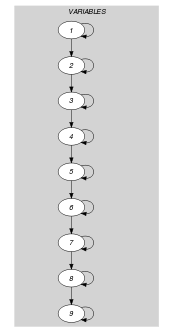(a) (b)

The $\mathrm{𝚐𝚛𝚘𝚞𝚙}$ constraint of the Example slot holds since:

• The final graph of the first graph constraint has two connected components. Therefore the number of groups $\mathrm{𝙽𝙶𝚁𝙾𝚄𝙿}$ is equal to two.

• The number of vertices of the smallest connected component of the final graph of the first graph constraint is equal to 1. Therefore $\mathrm{𝙼𝙸𝙽}_\mathrm{𝚂𝙸𝚉𝙴}$ is equal to 1.

• The number of vertices of the largest connected component of the final graph of the first graph constraint is equal to 2. Therefore $\mathrm{𝙼𝙰𝚇}_\mathrm{𝚂𝙸𝚉𝙴}$ is equal to 2.

• The number of vertices of the smallest connected component of the final graph of the second graph constraint is equal to 2. Therefore $\mathrm{𝙼𝙸𝙽}_\mathrm{𝙳𝙸𝚂𝚃}$ is equal to 2.

• The number of vertices of the largest connected component of the final graph of the second graph constraint is equal to 4. Therefore $\mathrm{𝙼𝙰𝚇}_\mathrm{𝙳𝙸𝚂𝚃}$ is equal to 4.

• The number of vertices of the final graph of the first graph constraint is equal to three. Therefore $\mathrm{𝙽𝚅𝙰𝙻}$ is equal to 3.

Automaton

Figures 5.172.35.172.55.172.85.172.105.172.12 and 5.172.14 depict the different automata associated with the $\mathrm{𝚐𝚛𝚘𝚞𝚙}$ constraint. For the automata that respectively compute $\mathrm{𝙽𝙶𝚁𝙾𝚄𝙿}$, $\mathrm{𝙼𝙸𝙽}_\mathrm{𝚂𝙸𝚉𝙴}$, $\mathrm{𝙼𝙰𝚇}_\mathrm{𝚂𝙸𝚉𝙴}$, $\mathrm{𝙼𝙸𝙽}_\mathrm{𝙳𝙸𝚂𝚃}$, $\mathrm{𝙼𝙰𝚇}_\mathrm{𝙳𝙸𝚂𝚃}$ and $\mathrm{𝙽𝚅𝙰𝙻}$ we have a 0-1 signature variable ${𝚂}_{i}$ for each variable ${\mathrm{𝚅𝙰𝚁}}_{i}$ of the collection $\mathrm{𝚅𝙰𝚁𝙸𝙰𝙱𝙻𝙴𝚂}$. The following signature constraint links ${\mathrm{𝚅𝙰𝚁}}_{i}$ and ${𝚂}_{i}$: ${\mathrm{𝚅𝙰𝚁}}_{i}\in \mathrm{𝚅𝙰𝙻𝚄𝙴𝚂}⇔{𝚂}_{i}$.

##### Figure 5.172.3. Automaton for the $\mathrm{𝙽𝙶𝚁𝙾𝚄𝙿}$ argument of the $\mathrm{𝚐𝚛𝚘𝚞𝚙}$ constraint and its glue matrix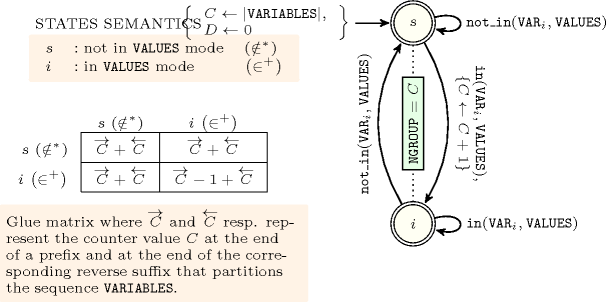##### Figure 5.172.4. Hypergraph of the reformulation corresponding to the automaton (with one counter) of the $\mathrm{𝙽𝙶𝚁𝙾𝚄𝙿}$ argument of the $\mathrm{𝚐𝚛𝚘𝚞𝚙}$ constraint (since all states of the automaton are accepting there is no restriction on the last variable ${Q}_{n}$)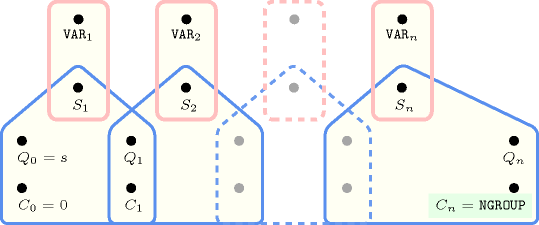##### Figure 5.172.5. Automaton for the $\mathrm{𝙼𝙸𝙽}_\mathrm{𝚂𝙸𝚉𝙴}$ argument of the $\mathrm{𝚐𝚛𝚘𝚞𝚙}$ constraint and its glue matrix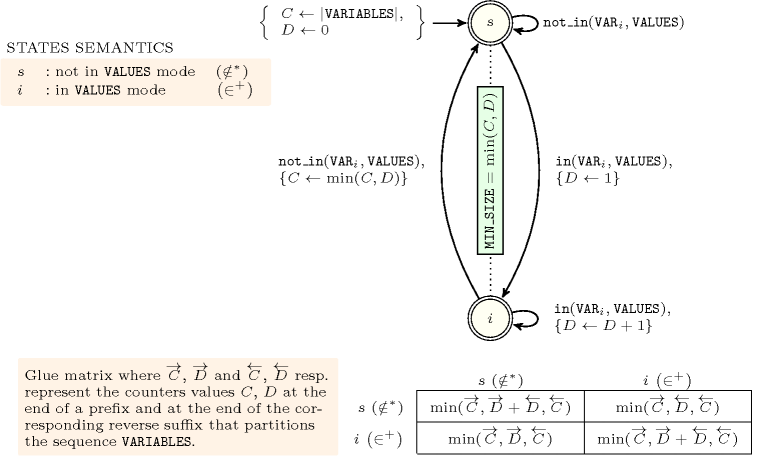##### Figure 5.172.6. Illustrating the use of the state pair $\left(i,i\right)$ of the glue matrix for linking $\mathrm{𝙼𝙸𝙽}_\mathrm{𝚂𝙸𝚉𝙴}$ with the counters variables obtained after reading the prefix $0,1,1,1,0,0,1$ and corresponding suffix $1,0,1,1,1,1$ of the sequence $0,1,1,1,0,0,1,1,0,1,1,1,1$; note that the suffix $1,0,1,1,1,1$ (in pink) is proceed in reverse order; the left (resp. right) table shows the initialisation (for $i=0$) and the evolution (for $i>0$) of the state of the automaton and its counters $C$ and $D$ upon reading the prefix $0,1,1,1,0,0,1$ (resp. the reverse suffix $1,1,1,1,0,1$).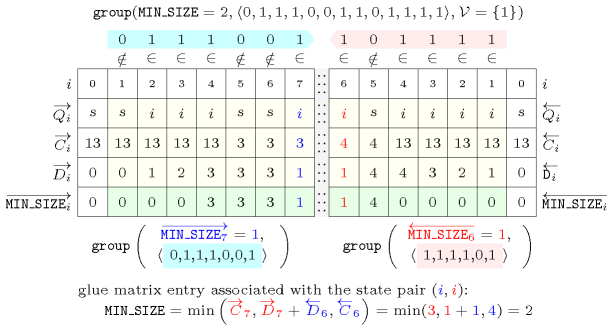##### Figure 5.172.7. Hypergraph of the reformulation corresponding to the automaton (with two counters) of the $\mathrm{𝙼𝙸𝙽}_\mathrm{𝚂𝙸𝚉𝙴}$ argument of the $\mathrm{𝚐𝚛𝚘𝚞𝚙}$ constraint where $𝙽$ stands for $|\mathrm{𝚅𝙰𝚁𝙸𝙰𝙱𝙻𝙴𝚂}|$ (since all states of the automaton are accepting there is no restriction on the last variable ${Q}_{n}$)##### Figure 5.172.8. Automaton for the $\mathrm{𝙼𝙰𝚇}_\mathrm{𝚂𝙸𝚉𝙴}$ argument of the $\mathrm{𝚐𝚛𝚘𝚞𝚙}$ constraint and its glue matrix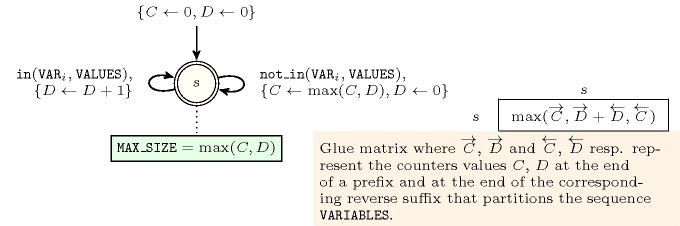##### Figure 5.172.9. Hypergraph of the reformulation corresponding to the automaton (with two counters) of the $\mathrm{𝙼𝙰𝚇}_\mathrm{𝚂𝙸𝚉𝙴}$ argument of the $\mathrm{𝚐𝚛𝚘𝚞𝚙}$ constraint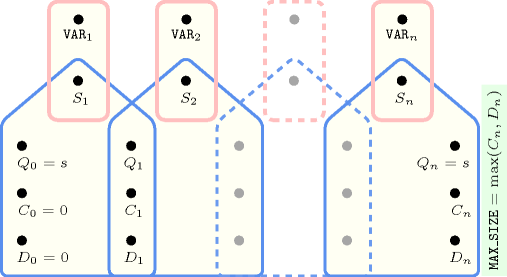##### Figure 5.172.10. Automaton for the $\mathrm{𝙼𝙸𝙽}_\mathrm{𝙳𝙸𝚂𝚃}$ argument of the $\mathrm{𝚐𝚛𝚘𝚞𝚙}$ constraint and its glue matrix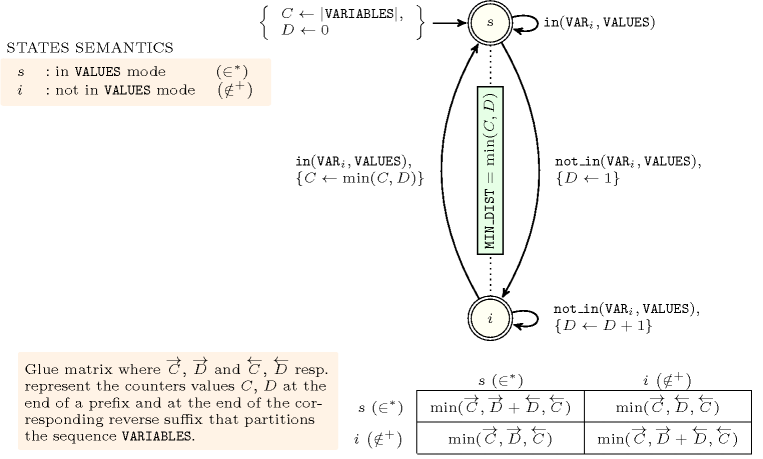##### Figure 5.172.11. Hypergraph of the reformulation corresponding to the automaton (with two counters) of the $\mathrm{𝙼𝙸𝙽}_\mathrm{𝙳𝙸𝚂𝚃}$ argument of the $\mathrm{𝚐𝚛𝚘𝚞𝚙}$ constraint where $𝙽$ stands for $|\mathrm{𝚅𝙰𝚁𝙸𝙰𝙱𝙻𝙴𝚂}|$ (since all states of the automaton are accepting there is no restriction on the last variable ${Q}_{n}$)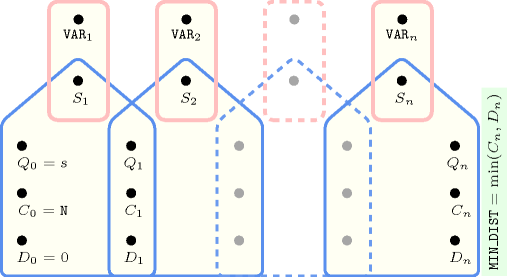##### Figure 5.172.12. Automaton for the $\mathrm{𝙼𝙰𝚇}_\mathrm{𝙳𝙸𝚂𝚃}$ argument of the $\mathrm{𝚐𝚛𝚘𝚞𝚙}$ constraint and its glue matrix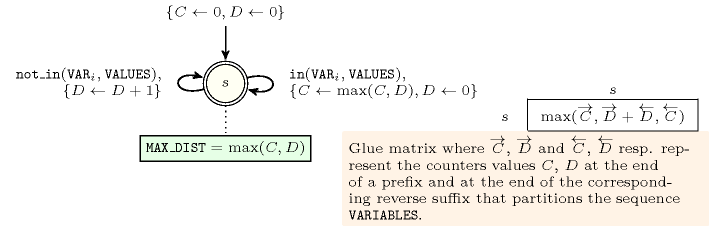##### Figure 5.172.13. Hypergraph of the reformulation corresponding to the automaton (with two counters) of the $\mathrm{𝙼𝙰𝚇}_\mathrm{𝙳𝙸𝚂𝚃}$ argument of the $\mathrm{𝚐𝚛𝚘𝚞𝚙}$ constraint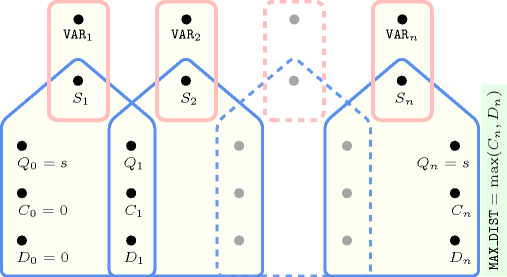##### Figure 5.172.14. Automaton for the $\mathrm{𝙽𝚅𝙰𝙻}$ argument of the $\mathrm{𝚐𝚛𝚘𝚞𝚙}$ constraint and its glue matrix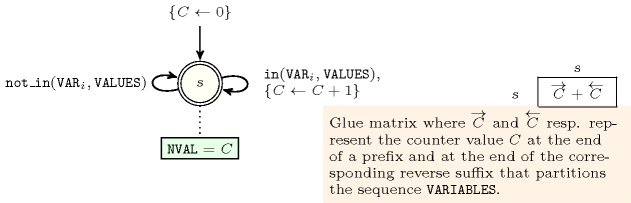##### Figure 5.172.15. Hypergraph of the reformulation corresponding to the automaton (with one counter) of the $\mathrm{𝙽𝚅𝙰𝙻}$ argument of the $\mathrm{𝚐𝚛𝚘𝚞𝚙}$ constraint